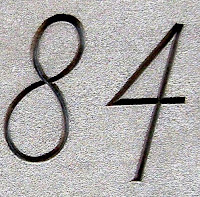## Friday, September 3, 2010

### 84

84 = 22 x 3 x 7.

84 is tetrahedral number, the sum of the first seven triangular numbers: 84 = 1 + 3 + 6 + 10 + 15 + 21 + 28.

84 has a unique representation as a sum of three squares: 84 = 22 + 42 + 82.

84 is the smallest number with eight representations as a sum of two primes: 84 = 5 + 79 = 11 + 73 = 13 + 71 = 17 + 67 = 23 + 61 = 31 + 53 = 37 + 47 = 41 + 43.

84 is 77 in base 11 and 66 in base 13.84 Charing Cross Road is a book by Helene Hanff.

Source: Phillips, R. 1994. Numbers: Facts, Figures and Fiction. Cambridge University Press.